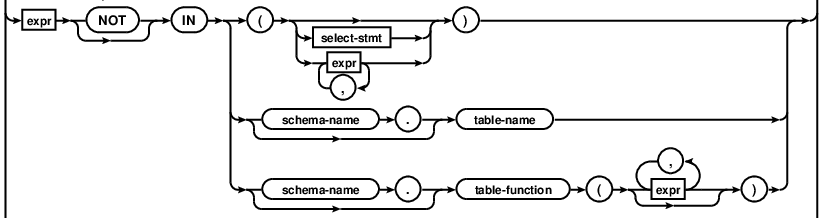# Sqlite IN clause 小常識

Query 大概長這樣：

`SELECT * FROM some_table         --- AWHERE some_column IN ((  SELECT * FROM another_table    --- B));`expr 中的 IN 的部份

`sqlite> CREATE TABLE temp (   ...> id integer   ...> );sqlite> INSERT INTO temp VALUES(1),(2),(3);sqlite> SELECT * FROM temp WHERE id IN (1,2,3);   id = 1   id = 2   id = 3sqlite> SELECT * FROM temp WHERE id IN (SELECT id FROM temp);   id = 1   id = 2   id = 3sqlite> SELECT * FROM temp WHERE id IN ((SELECT id FROM temp));   id = 1sqlite> SELECT * FROM temp WHERE id IN ((SELECT id FROM temp), (SELECT 2 FROM temp));   id = 1   id = 2`